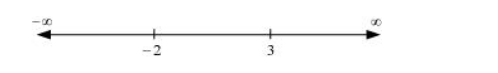# Find the intervals in which the function

Question:

Find the intervals in which the function $f$ given by $f(x)=2 x^{3}-3 x^{2}-36 x+7$ is

(a) strictly increasing (b) strictly decreasing

Solution:

The given function is $f(x)=2 x^{3}-3 x^{2}-36 x+7$.

$f^{\prime}(x)=6 x^{2}-6 x-36=6\left(x^{2}-x-6\right)=6(x+2)(x-3)$

$\therefore f^{\prime}(x)=0 \Rightarrow x=-2,3$

The points $x=-2$ and $x=3$ divide the real line into three disjoint intervals i.e.,

$(-\infty,-2),(-2,3)$, and $(3, \infty)$In intervals $(-\infty,-2)$ and $(3, \infty), f^{\prime}(x)$ is positive while in interval

$(-2,3), f^{\prime}(x)$ is negative.

Hence, the given function (f) is strictly increasing in intervals

$(-\infty,-2)$ and $(3, \infty)$, while function $(f)$ is strictly decreasing in interval

(−2, 3).